# Proton 5: 3rd Degree, Polar Star (P5-35P)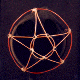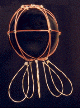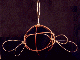Oh man! Did I have to work to find this one! Boron was kind of a holy grail for me, I spent years puzzling over it. For many years the only solutions I had were P1, N1, P2, N2. All of them had features in common... all the quarks were equidistant on the surface of the shell, and all of them could be solved with two loop sizes. I falsely believed that all nuclear shells would have their quarks spaced equidistant, and I just could not find such a solution for boron. I knew it was special in several ways, and I was sure that finding a solution for the fifteen quark shells of boron would be the key to understanding nuclear structure. I studied the boron hydrides, planar molecules folded on themselves by charge. Boron is a semiconductor, and I was hoping to confirm a metallic bonding model using its nuclear structure as a guide. I just couldn't get it though, and I was certain that two different isotopes of boron were involved in the boron hydrides. At the very least, radical strings were in different pinning sites. The 3rd degree solution for P5 has a startling number of radical strings, five of them. There are no genuine down quarks on the 3rd degree P5, only bogus down quarks. (The 4th degree solution offers only two radical strings.) The first photo shows P5 stripped of radical strings, the remaining photos show two possible pinning configurations, but there are many more. The neutron shell will ultimately decide the final pinning points, but since boron typically has five or six neutrons, the final stacking solutions should still have some flexibility in their pinning sites.

## Stereograms of P5-35P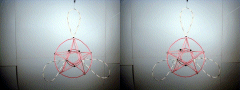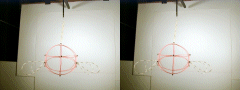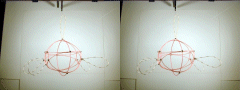Required Snap Points: 50 Available Snap Points: 45 Pinned Vector Bosons: 5 L1 = 37.2007141" (Structural) L2 = 39.11514559" (Equatorial) L3 = 14.94064195" (Polar) Model Diameter: 12.45073754" Loop Ratios: (1:2): 0.951056516 (1:3): 2.48990065 (2:3): 2.618036475 Loop Equation: 5(L1) + 1(L2) + 2(L3) = 255" Snap Point Equation: 5(6) + 1(5) + 2(5) = 45 A.S.P. PVB's:(+ 5 P.V.B. = 50 R.S.P.)

# Proton 5: 3rd Degree, Polar Star (P5-30PS)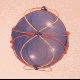This is also a third degree solution which uses a sinusoidal-equatorial string wrapping twice around the particle much like the P1 and N1 shells. All the down quarks are located at the equator, and no radical strings are required.

## Stereograms of P5-30PS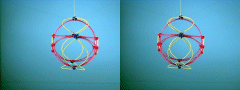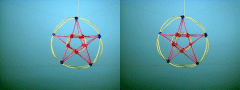Required Snap Points: 50 Available Snap Points: 50 Pinned Vector Bosons: 0 L1 = 28.6729006" (Structural) L2 = 88.6041357" (Sinusoidal-Equatorial) L3 = 11.5156806" (Polar) Model Diameter: 9.596556646" Loop Ratios: (1:2): 0.333333333 (1:3): 2.48990065 (2:3): 7.694216149 Loop Equation: 5(L1) + 1(L2) + 2(L3) = 255" Snap Point Equation: 5(6) + 1(10) + 2(5) = 50 R.S.P.

# Proton 5: 4th Degree, Polar Star (P5-42P)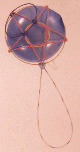This fourth degree solution for P5 pins two radical strings. I have successfully stacked this shell with (N5-41P) to form a B10 (Boron) nucleus with a complementary shell structure, and have further identified it as a valid solution set from studies of the boron hydrides and predictions made in my 1989 paper "Time and Geometry." But please, don't take my word for it, go verify it yourself, after all, that's why this web site exists. The pinning sites in the stacked model differ from what is shown here, but recall that I pin the strings for individual shells in a way that best balances their charge structure. Actually stacking the shells is the only way to determine the appropriate pinning sites for vector bosons (radical strings).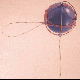## Stereograms of P5-42P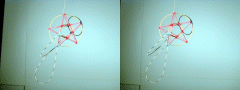Required Snap Points: 50 Available Snap Points: 48 Pinned Vector Bosons: 2 L1 = 34.07118437" (Structural) L2 = 35.82456329" (Equatorial) L3 = 13.68375255" (Polar) L4 = 21.45200976" (Filler) Model Diameter: 11.40331266" Loop Ratios: (1:2): 0.951056516 (1:3): 2.48990065 (1:4): 1.588251392 (2:3): 2.618036475 (2:4): 1.669986341 (3:4): 0.637877416 Loop Equation: 5(L1) + 1(L2) + 2(L3) + 1(L4) = 255" Snap Point Equation: 5(6) + 1(5) + 2(5) + 1(3) = 48 A.S.P. PVB's:(+ 2 P.V.B. = 50 R.S.P.)

Copyright 1997 by Arnold J. Barzydlo
Go Back